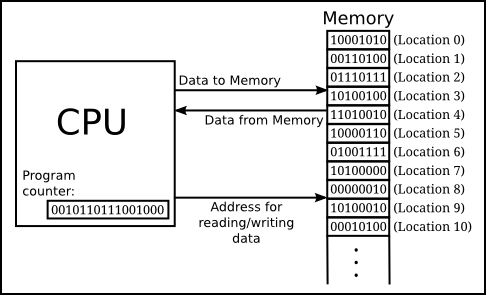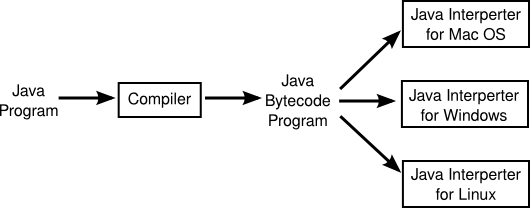# Course Introduction

## Algorithms

Computer science is the study of Algorithms.

An algorithm is a precise, step by step procedure for solving some problem in general.

Algorithms:

• Predate computers by thousands of years.
• Can be followed without understanding.

In this course we will create and analyze algorithms for a variety of problems.

## Computers

Computers are essentially very simple machines for executing algorithms automatically. Computers consist of a central processing unit (CPU), which is capable of performing math on numbers, and a memory, which stores numbers in binary:## Programs

A computer program is an algorithm that is written in a language that a computer can understand. Programming lets us test and run our algorithms easily.

Computers only natively understand binary machine code. These are essentially numbers which are encoded to refer to instructions for the computer to carry out. For example, the instruction:

00000001101110100100100000100000

Might tell a computer to add the bits in two memory locations, and put the result in a third.

As writing programs in this way is quite tedious, we have developed high-level programming languages which allow for a more easily understood form. For example, to perform an addition we could write:

x = y + z;

All high-level programming languages must have their code converted into machine code in some way.

## Java

Java is a programming language created in 1995 by Sun Microsystems, and is now maintained by Oracle. Java programs are first compiled into Java bytecode files which are executed by the Java Virtual Machine (JVM).

Java is very widely used including:

• Websites such as Amazon and Ebay.
• Most Android applications.
• Games such as Runescape and Minecreaft.

Java uses compiler and a virtual machine to convert the Java code into machine instructions:The compiler converts the Java code into "bytecode" which is a form of machine code which is portable, and not specific to any real computer. This bytecode is then executed by a Java virtual machine (JVM) which converts the bytecode into the native machine code for your particular computer.

## Hello World

The simplest program is one that prints "Hello world!" to the user. The Java version of Hello World appears below:


public class Hello {
public static void main(String args[]) {
System.out.println("Hello World!");
}
}